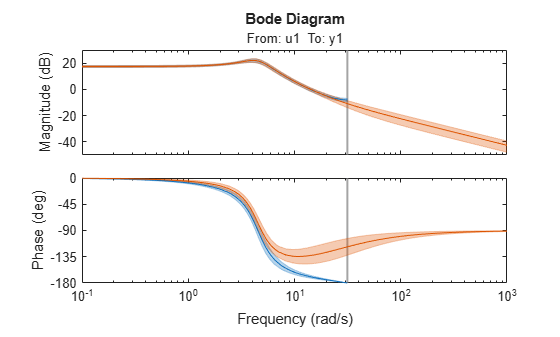d2c

Convert model from discrete to continuous time

Description

example

sysc = d2c(sysd) converts a the discrete-time dynamic system model sysd to a continuous-time model using zero-order hold on the inputs.

example

sysc = d2c(sysd,method) specifies the conversion method.

example

sysc = d2c(sysd,opts) specifies conversion options for the discretization.

[sysc,G] = d2c(___), where sysd is a state-space model, returns a matrix G that maps the states xd[k] of the discrete-time state-space model to the states xc(t) of sysc.

Examples

collapse all

Create the following discrete-time transfer function:

$H\left(z\right)=\frac{z-1}{{z}^{2}+z+0.3}$

H = tf([1 -1],[1 1 0.3],0.1);

The sample time of the model is ${T}_{s}=0.1s$.

Derive a continuous-time, zero-order-hold equivalent model.

Hc = d2c(H)
Hc =

121.7 s + 2.904e-12
---------------------
s^2 + 12.04 s + 776.7

Continuous-time transfer function.

Discretize the resulting model, Hc, with the default zero-order hold method and sample time 0.1s to return the original discrete model, H.

c2d(Hc,0.1)
ans =

z - 1
-------------
z^2 + z + 0.3

Sample time: 0.1 seconds
Discrete-time transfer function.

Use the Tustin approximation method to convert H to a continuous time model.

Hc2 = d2c(H,'tustin');

Discretize the resulting model, Hc2, to get back the original discrete-time model, H.

c2d(Hc2,0.1,'tustin');

Estimate a discrete-time transfer function model, and convert it to a continuous-time model.

sys1d = tfest(z1,2,'Ts',0.1);
sys1c = d2c(sys1d,'zoh');

Estimate a continuous-time transfer function model.

sys2c = tfest(z1,2);

Compare the response of sys1c and the directly estimated continuous-time model, sys2c.

compare(z1,sys1c,sys2c)The two systems are almost identical.

Convert an identified discrete-time transfer function model to continuous-time.

sysd = tfest(z1,2,'Ts',0.1);
sysc = d2c(sysd,'zoh');

sys1c has no covariance information. The d2c operation leads to loss of covariance data of identified models.

Regenerate the covariance information using a zero iteration update with the same estimation command and estimation data.

opt = tfestOptions;
opt.SearchOptions.MaxIterations = 0;
sys1c = tfest(z1,sysc,opt);

Analyze the effect on frequency-response uncertainty.

h = bodeplot(sysd,sys1c);
showConfidence(h,3)The uncertainties of sys1c and sysd are comparable up to the Nyquist frequency. However, sys1c exhibits large uncertainty in the frequency range for which the estimation data does not provide any information.

If you do not have access to the estimation data, use the translatecov command which is a Gauss-approximation formula based translation of covariance across model type conversion operations.

Input Arguments

collapse all

Discrete-time model, specified as a dynamic system model such as idtf, idss, or idpoly.

You cannot directly use an idgrey model whose FunctionType is 'd' with d2c. Convert the model into idss form first.

Discrete-to-continuous time conversion method, specified as one of the following values:

• 'zoh' — Zero-order hold on the inputs. Assumes that the control inputs are piecewise constant over the sampling period.

• 'foh' — Linear interpolation of the inputs (modified first-order hold). Assumes that the control inputs are piecewise linear over the sampling period.

• 'tustin' — Bilinear (Tustin) approximation to the derivative. To specify this method with frequency prewarping (formerly known as the 'prewarp' method), use the PrewarpFrequency option of d2cOptions.

• 'matched' — Zero-pole matching method (for SISO systems only). See  .

For information about the algorithms for each d2c conversion method, see Continuous-Discrete Conversion Methods.

Discrete-to-continuous time conversion options, created using d2cOptions. For example, specify the prewarp frequency or the conversion method as an option.

Output Arguments

collapse all

Continuous-time model, returned as a dynamic system model of the same type as the input system sysd.

When sysd is an identified (IDLTI) model, sysc:

• Includes both the measured and noise components of sysd. If the noise variance is λ in sysd, then the continuous-time model sysc has an indicated level of noise spectral density equal to Ts*λ.

• Does not include the estimated parameter covariance of sysd. If you want to translate the covariance while converting the model, use translatecov.

Mapping of the states xd[k] of the state-space model sysd to the states xc(t) of sysc, returned as a matrix. The mapping of the states is as follows:

${x}_{c}\left(k{T}_{s}\right)=G\left[\begin{array}{c}{x}_{d}\left[k\right]\\ u\left[k\right]\end{array}\right].$

Given an initial condition x0 for sysd and an initial input u0 = u, the corresponding initial condition for sysc (assuming u[k] = 0 for k < 0 is:

${x}_{c}\left(0\right)=G\left[\begin{array}{c}{x}_{0}\\ {u}_{0}\end{array}\right].$

 Franklin, G.F., Powell,D.J., and Workman, M.L., Digital Control of Dynamic Systems (3rd Edition), Prentice Hall, 1997.

 Kollár, I., G.F. Franklin, and R. Pintelon, "On the Equivalence of z-domain and s-domain Models in System Identification," Proceedings of the IEEE® Instrumentation and Measurement Technology Conference, Brussels, Belgium, June, 1996, Vol. 1, pp. 14-19.

System Identification Toolbox DocumentationGet trial now